# How to Find and Replace Wildcards like Asterisk/Question Mark/Tilde in Excel

We may enter some special characters like Asterisk (*)/Question Mark(?)/Tilde (~) in excel, and these special characters are recognized as wildcards when finding and replacing them in excel. So, if we want to find or replace the wildcards with the others, we may get the improper result or the result is not the result we expected. This article will provide you the method to find and replace wildcards in excel, let’s follow below steps to learn it.

First see an example: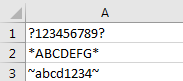Asterisk/Question Mark/Tilde are included in contents. If we find or replace these characters with the others what result we will get?

Find` ‘?’` in above table.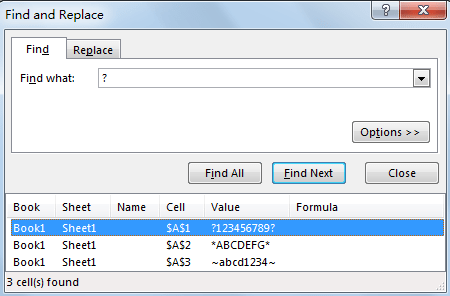Notice that if we enter ‘?’ in ‘Find what’ textbox and click ‘Find All’ in ‘Find and Replace’ screen, all cells with contents are listed in result.

Replace `*` with OK.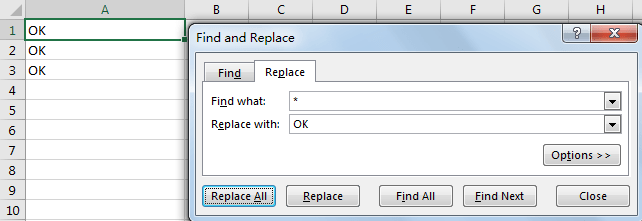Notice that the completed contents are replaced by OK. So how can we handle this case? Please see the following steps to get the solution.

## Find or Replace Wildcards like Asterisk/Question Mark/Tilde by Add ~ before Them in Excel

1.  Click Home->Find & Selection->Find (or Replace) to enter Find and Replace screen.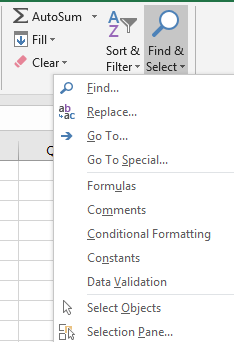2. Enter `~?` in Find what textbox.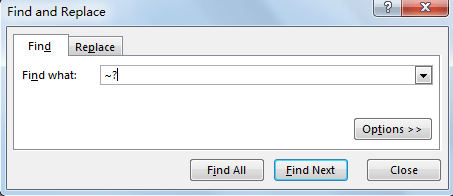3. Click Find All to load result. Verify that only the cell includes ? is loaded. The others are not listed in the result list.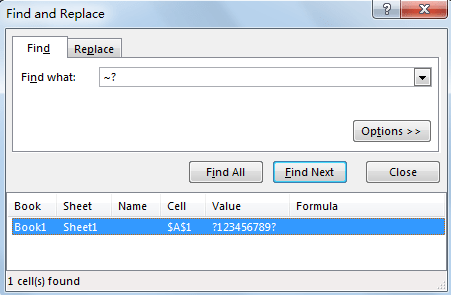4. Replace * with OK. Click on Replace tab. You can also click Ctrl+H to load Find and Replace screen directly. Enter ~* in Find what textbox.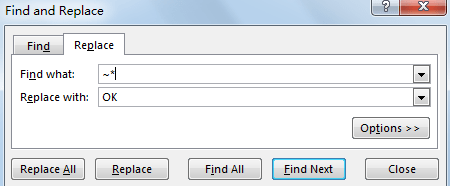5. Click Replace All. Verify that “All done. We made two replacements.” dialog box pops up.

6. Check the result. Verify that * is replaced with OK properly now.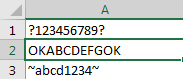7. The same operation can be used for find and replace ~. We just need to enter ~~ in textbox to do find and replace.

Related Posts

Calculate Number of Hours between Two Times

Calculating the difference between two times might be a valuable statistic for subsequent computations or averages, whether you're producing a time sheet for staff or recording personal exercises. While Excel has a plethora of complex functions, including date and time ...

Calculate Loan Interest in Given Year

When you borrow money, you are supposed to repay it gradually. Lenders, on the other hand, want to be compensated for their services and the risk they incur by lending you money. That is, you will not just repay the ...

Calculate Interest Rate for Loan

The interest rate is the fee charged by a lender to a borrower and is expressed as a percentage of the principal—the lent amount. The interest rate on a loan is often expressed as an annual percentage rate, abbreviated as ...

Calculate Interest for Given Period

Using the IPMT function in Excel, we can compute the interest payment on any loan. This step-by-step tutorial will guide Excel users of all skill levels through the process to calculate interest for given period. Finally, the formula: =IPMT(B3/12,1,B5,-B2) The ...

How To Use Excel GCD Function

This post will guide you how to use Excel GCD function with syntax and examples in Microsoft excel. Description The Excel GCD function Returns the greatest common divisor of two or more integers. So you can use the GCD function ...

Calculate A Ratio From Two Numbers In Excel

In elementary mathematics, a ratio is a connection or comparison between two or more integers. For example, ratios are often expressed as ":" to demonstrate the relationship between two numbers. You would think that manually calculating a ratio from two ...

How To Use Excel RRI Function

This post will guide you how to use Excel RRI function with syntax and examples in Microsoft excel. Description The Excel RRI function Returns an equivalent interest rate for the growth of an investment. So you can use the RRI ...

CAGR Formula Examples in Excel

CAGR in Excel is a formula that calculates the compound annual growth rate for any invested amount over the specified years or timeframe. Although there is no direct function in Excel that can help us identify the CAGR value, there ...

Build Hyperlink With VLOOKUP in Excel

You might have come across a task in which you were assigned to build hyperlinks, which seems very easy, and if you are new to excel or don't have enough experience with it, then you might wonder about doing this ...

Break ties with helper COUNTIF and column

Suppose you got a task to adjust the values that contain the ties; what would be your first attempt to break the ties of the given value? If you are wondering about doing this task manually, let me add that ...

Sidebar